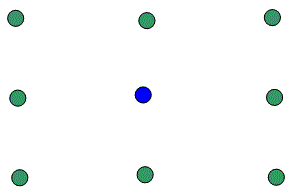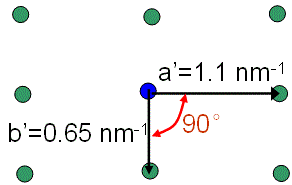Indexing Electron Diffraction Patterns Starting with Zone Axis
- Practical Electron Microscopy and Database -
- An Online Book -
Microanalysis | EM Book                                                                                   http://www.globalsino.com/EM/

This book (Practical Electron Microscopy and Database) is a reference for TEM and SEM students, operators, engineers, technicians, managers, and researchers.
=================================================================================

As mentioned in page4825, in general, indexing electron diffraction patterns is an empirical work with theoretical understanding. Assuming we have an electron diffraction pattern shown in Figure 3090a, and we know the crystal is an fcc structure.Figure 3090a. An fcc diffraction pattern for indexing.

Then, we can index it in following steps:

i) Measure the reciprocal lattice spacings and the angle between the basis vectors a' and b' as shown in Figure 3090b. These measurements can be done on the user interface (UI) of the microscope, e.g. Gatan Digital Micrograph.Figure 3090b. Measurements of the reciprocal lattice spacings and the angle between the basis vectors a' and b'.

ii) The easiest way is to index it by referring to the Standard Indexed Diffraction Patterns for fcc Crystals on page3915. Note that for other structures, we can also find the relevant standard diffraction patterns at link. Otherwise, we can more generally index the pattern by skipping this step and performing the next step.

iii) We notice that the pattern in Figure 3090a is very likely a low-order zone axis as the density of spots is high. Then, the lowest order zone axes will be our first try.

iv) List all the lowest order zone axes for fcc structure:
  
    
 

v) We know three directions above (, , and ) are the same, so that we need only consider the lowest index  direction as a possible zone axis and can narrow down the list:
  
   


vi) The ,  and  zone axes are further ignored because their symmetries (see diffraction patterns for FCC) does not match that of the analyzing diffraction pattern in Figure 3090a. Therefore, we narrow down the list again:
   


vii) Since there are not many candidates left, we can start to guess and test our guess. First, we test  zone axis. The allowed diffractions from fcc structures should have h, k, l are all even or all odd (see page3560), so that we list the lowest order diffractions in the  diffraction pattern below:
(111) (200) (220) (311) (331) (420)
(222) (400)
(333)

viii) In this case, as shown in Figure 3090a, the allowed diffraction spots must be perpendicular to the  zone axis. To test it, their dot products need to be calculated. We can only expect the lowest order spots are (002) and (2-40) because of · = 0 and ·[2-40] = 0. However, the others are: · ≠ 0, · ≠ 0, · ≠ 0, and · ≠ 0.

ix) Verify if the angle between the two vectors running from the (000) spot to these two specific diffraction spots is correct (here is for FCC). This is consistent with the 90° angle on the diffraction pattern (see Figure 3090c) as their dot product is exactly zero (i.e. ·[2-40] = 0). Note that the angles for different crystal structures can be calculated using the relevant equations in page3086.Figure 3090c. Angle between the two vectors running from the (000) spot to these two diffraction spots.

Once we have measured the values for g1 and g2, we need to cross-check our answers using the angles between the g vectors. Note that, in general, we do not need to measure more than two or three spacings for indexing a diffraction pattern.

x) Based on the camera length theory (see page3580), we should have,---------------------------------------- [3090a]
where,
Rh1,k1,l1 and Rh2,k2,l2 -- The reciprocal lattice spacings, measured as the distances from the origin (O, the location of the directly transmitted beam on the screen) to the two diffraction spots in Figure 3090c.

However, based on the numbers in Figures 3090b and 3090c, we actually have,---------------------- [3090b]
Therefore, the  zone axis is not correct, and thus we narrow down the list (on step vi) again:
  


xi) We need to re-guess another zone axis, e.g.  zone axis. Similar to step viii, the allowed diffraction spots must be perpendicular to the  zone axis. The calculated dot products are: ·[-111] = 0, · ≠ 0, ·[02-2] = 0, ·[11-3] = 0, · ≠ 0, ·[2-40] = 0. Then, the indexed pattern is shown in Figure 3090d.Figure 3090d. Angle between the two vectors running from the (000) spot to these two diffraction spots.

xii) Based on the numbers in Figures 3090b and 3090d, we have,------------------- [3090c]
Therefore, we can say that we have gotten the correct indexing with a reasonable inaccuracy, which can be caused by the measurement of the dimensions or there may be some distortion of the diffraction spots.

=================================================================================
The book author (Yougui Liao) welcomes your comments, suggestions, and corrections, please click here for submission. If you let book author know once you have cited this book, the brief information of your publication will appear on the “Times Cited” page.